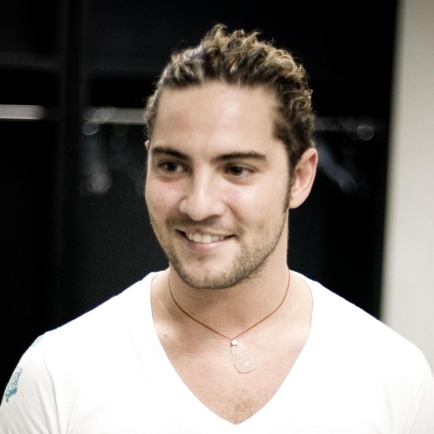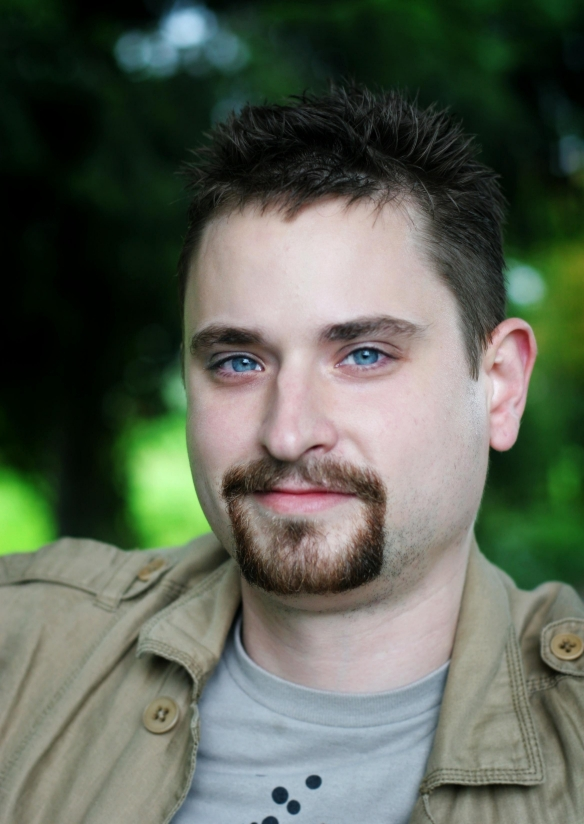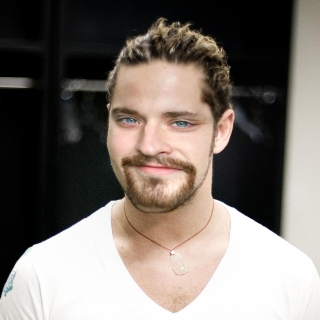OpenCV  4.0.0 Open Source Computer Vision
Face swapping using face landmark detection

This application lets you swap a face in one image with another face in other image. The application first detects faces in both images and finds its landmarks. Then it swaps the face in first image with in another image. You just have to give paths to the images run the application to swap the two faces.

// Command to be typed for running the sample

### Description of command parameters

• image1 i1 (REQUIRED) Path to the first image file in which you want to apply swapping.
• image2 i2 (REQUIRED) Path to the second image file in which you want to apply face swapping.
• model m (REQUIRED) Path to the file containing model to be loaded for face landmark detection.
• face_cascade f (REQUIRED) Path to the face cascade xml file which you want to use as a face detector.

### Understanding the code

This tutorial will explain the sample code for face swapping using OpenCV. Jumping directly to the code :

bool myDetector( InputArray image, OutputArray ROIs );
bool myDetector( InputArray image, OutputArray ROIs ){
Mat gray;
std::vector<Rect> faces;
if(image.channels()>1){
cvtColor(image.getMat(),gray,COLOR_BGR2GRAY);
}
else{
gray = image.getMat().clone();
}
equalizeHist( gray, gray );
face_cascade.detectMultiScale( gray, faces, 1.1, 3,0, Size(30, 30) );
Mat(faces).copyTo(ROIs);
return true;
}

The facemark API provides the functionality to the user to use their own face detector to be used in face landmark detection.The above code creartes a sample face detector. The above function would be passed to a function pointer in the facemark API.

FacemarkKazemi::Params params;
params.configfile = configfile_name;
Ptr<Facemark> facemark = FacemarkKazemi::create(params);
facemark->setFaceDetector(myDetector);

The above code creates a pointer of the face landmark detection class. The face detector created above has to be passed as function pointer to the facemark pointer created for detecting faces while training the model.

vector<Rect> faces1,faces2;
vector< vector<Point2f> > shape1,shape2;
float ratio1 = (float)img1.cols/(float)img1.rows;
float ratio2 = (float)img2.cols/(float)img2.rows;
resize(img1,img1,Size(640*ratio1,640*ratio1),0,0,INTER_LINEAR_EXACT);
resize(img2,img2,Size(640*ratio2,640*ratio2),0,0,INTER_LINEAR_EXACT);
Mat img1Warped = img2.clone();
facemark->getFaces(img1,faces1);
facemark->getFaces(img2,faces2);
facemark->fit(img1,faces1,shape1);
facemark->fit(img2,faces2,shape2);

The above code creates vectors to store the detected faces and a vector of vector to store shapes for each face detected in both the images.It then detects landmarks of each face detected in both the images.the images are resized as it is easier to process small images. The images are resized according their actual ratio.

vector<Point2f> boundary_image1;
vector<Point2f> boundary_image2;
vector<int> index;
convexHull(Mat(points2),index, false, false);
for(size_t i = 0; i < index.size(); i++)
{
boundary_image1.push_back(points1[index[i]]);
boundary_image2.push_back(points2[index[i]]);
}

The above code then finds convex hull to find the boundary points of the face in the image which has to be swapped.

vector< vector<int> > triangles;
Rect rect(0, 0, img1Warped.cols, img1Warped.rows);
divideIntoTriangles(rect, boundary_image2, triangles);
for(size_t i = 0; i < triangles.size(); i++)
{
vector<Point2f> triangle1, triangle2;
for(int j = 0; j < 3; j++)
{
triangle1.push_back(boundary_image1[triangles[i][j]]);
triangle2.push_back(boundary_image2[triangles[i][j]]);
}
warpTriangle(img1, img1Warped, triangle1, triangle2);
}

Now as we need to warp one face over the other and we need to find affine transform. Now as the function in OpenCV to find affine transform requires three set of points to calculate the affine matrix. Also we just need to warp the face instead of the surrounding regions. Hence we divide the face into triangles so that each triiangle can be easily warped onto the other image.

The function divideIntoTriangles divides the detected faces into triangles. The function warpTriangle then warps each triangle of one image to other image to swap the faces.

vector<Point> hull;
for(size_t i = 0; i < boundary_image2.size(); i++)
{
Point pt((int)boundary_image2[i].x,(int)boundary_image2[i].y);
hull.push_back(pt);
}
Mat mask = Mat::zeros(img2.rows, img2.cols, img2.depth());
Rect r = boundingRect(boundary_image2);
Point center = (r.tl() + r.br()) / 2;
Mat output;
img1Warped.convertTo(img1Warped, CV_8UC3);
imshow("Face_Swapped", output);

Even after warping the results somehow look unnatural. Hence to improve the results we apply seamless cloning to get the desired results as required.

### Results

Consider two images to be used for face swapping as follows :

## First image## Second image## Results after swapping